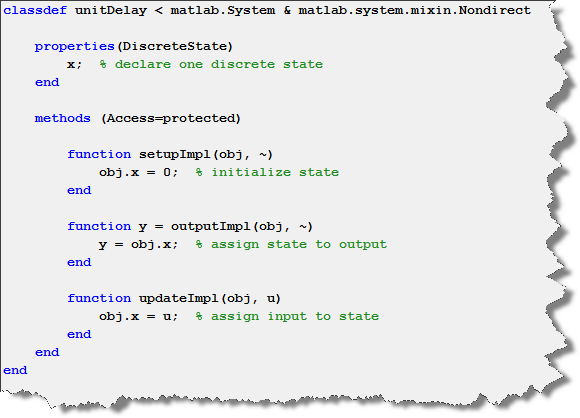# The MATLAB System Block

In R2013b, you will notice a new block in the Simulink Library Browser: The MATLAB System block.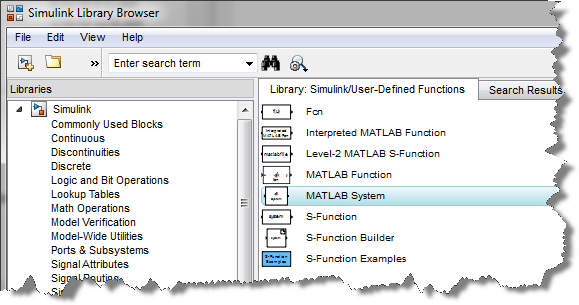System Object

Before looking at the MATLAB System block, let's looks at the evolution of System objects.

In R2010a, System objects were introduced in the Signal Processing Blockset. In contrast to MATLAB functions, System objects are object-oriented implementations of algorithms that can automatically manage state information, data indexing, and buffering, which is particularly useful in digital signal processing.

In R2011a, System toolboxes were introduced. Built on System objects, those toolboxes give you the possibility to design (in MATLAB or Simulink) signal processing algorithms suitable for fast simulation, real-time prototyping, and embedded implementation.

Starting in R2011b, users can create custom System objects in MATLAB. To include those System objects in Simulink, the MATLAB Function block could be used, but it required some tricks (as shown in this post).

In R2013b the MATLAB System block makes it very easy to use custom System objects in Simulink.

A Simple Example

To illustrate the concept of using a System object in MATLAB and Simulink, I made a timesTwo System object based on the AddOne example included the documentation.

For that, all I need is to create a new class file, which inherits from the matlab.System class. Then I put the equations for my block in the stepImpl method: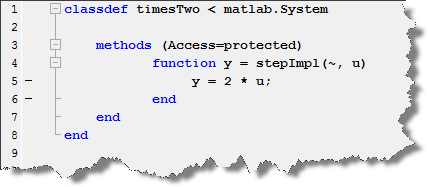I can use this System object in MATLAB with just two lines of code: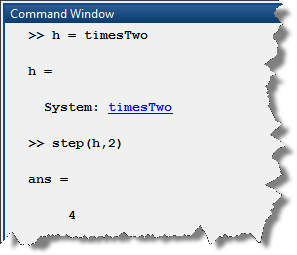In Simulink, it is as simple a dragging the block, and specifying the name of the object: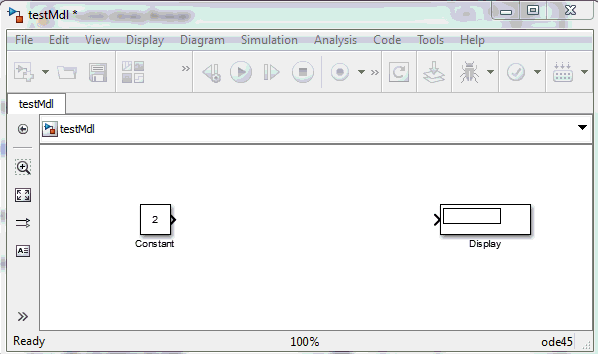Why do we need a MATLAB System block?

The previous example is too simple to highlight the power of the MATLAB System block. With System objects, you will find a large number of methods to setup the properties of states, output signals and input signals. You will even find methods to manage the MATLAB System block icon and dialog.

In the next weeks, I will follow up with a series of posts on the MATLAB System block to highlight more of its capabilities. For today, I will leave you with an example of a unit delay. When using the code below in the MATLAB System block, the input is marked as non-directfeedthrough. This makes it possible to use this block in loop without creating an algebraic loop.